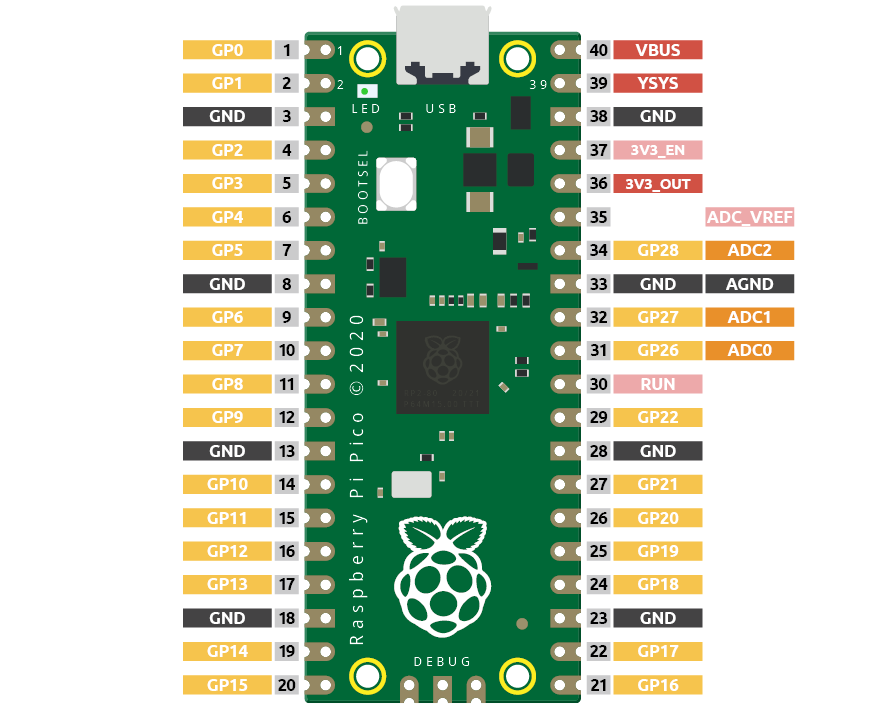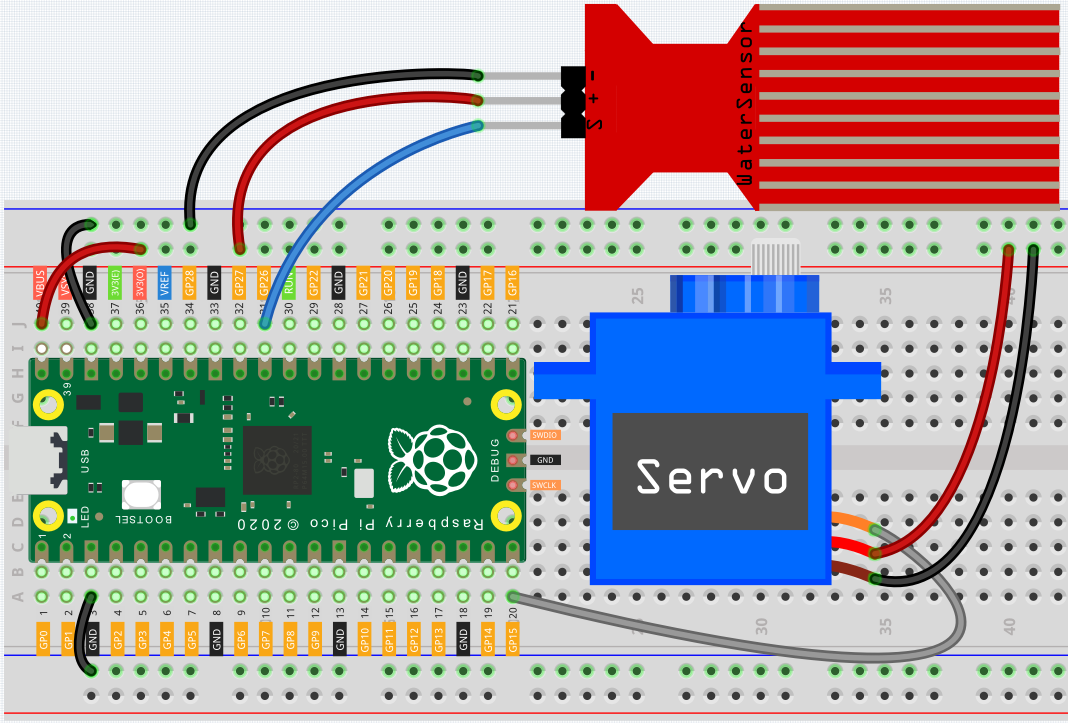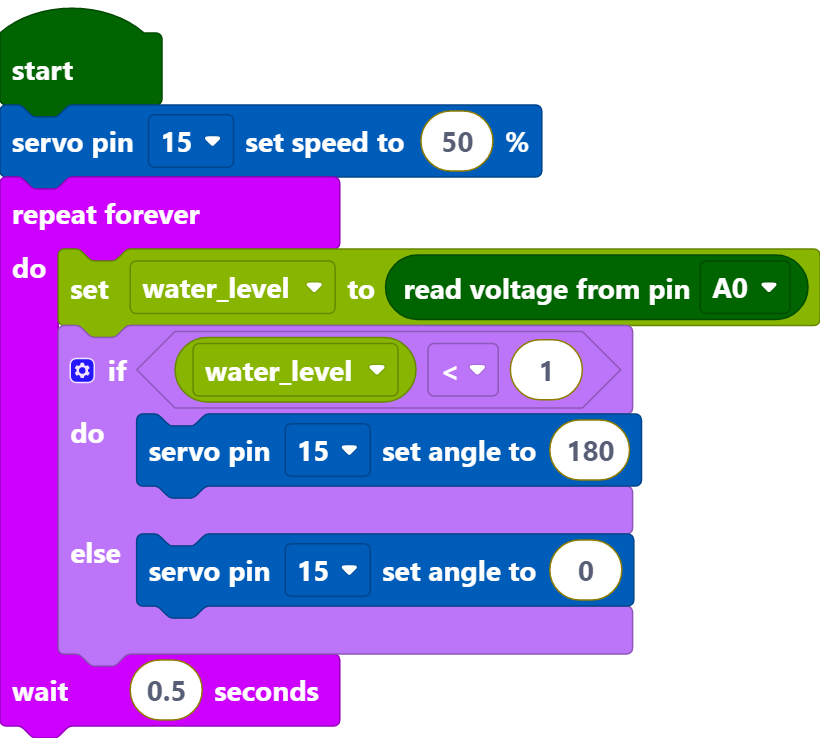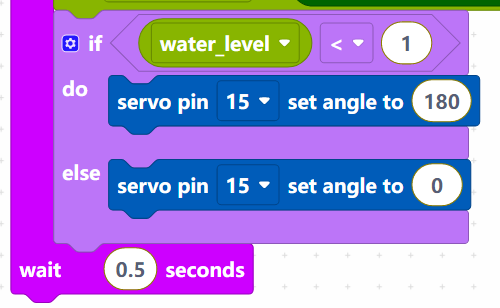# 2.6 Smart Water Tank¶

In this project, we use a water level sensor module and a servo to simulate a smart water tank. The water level sensor is fixed inside the tank to measure the water level, and when the water level is below a threshold, the valve controlled by the servo is allowed to open to let water in.

WiringPico has three GPIO pins that can use analog input, GP26, GP27, GP28. That is, analog channels 0, 1, and 2. In addition, there is a fourth analog channel, which is connected to the built-in temperature sensor and will not be introduced here.• S of water level sensor is connected to GP26(A0), + to VBUS, - pin to GND.

• The orange wire (signal) of the servo is connected to GP15, the red wire (power) is connected to VBUS, and the brown wire (ground) is connected to GND.

Code

Note

• You can refer to the image below to write code by dragging and dropping.

• Import `2.6_water_tank.png` from the path of `euler-kit\piper`. For detailed tutorials, please refer to Import the Code.After connecting Pico, click the Start button and the code starts to run. When the water level is lower than 1/3 of the water level sensor, the servo rotates to 180 degrees to simulate the inlet opening; when the water level is higher than 1/3 of the water level sensor, the servo rotates to 0 degrees to imitate the inlet closing.

How it Works?Set the rotation speed of pin15 (servo) to 15%.

• [servo pin() set speed to ()%]：Used to set the rotation speed of the servo pin, the range is 0%~100%.Reads the value of pin A0 and stores it in the variable [water_level].

• [set (water_level) to]: Used to set the value of the variable, you need to create the variable from the Variables palette.

• [read voltage from pin ()]: Used to read the voltage of the analog pins (A0~A2), the range is 0 ~ 3.3V.Set the voltage threshold to 1. When the voltage of water level sensor is less than 1, let the servo rotate to 180° position, otherwise rotate to 0° position.

• [servo pin () set angle to ()]: Set the angle of servo pin to, the range is 0 ~ 180°.